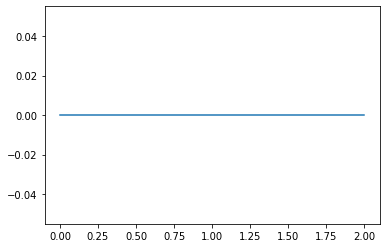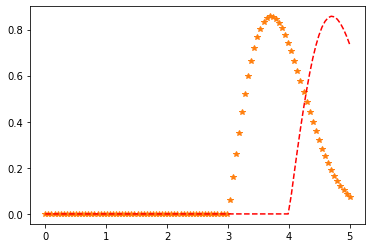Python – Nakagami Distribution in Statistics

• Last Updated : 10 Jan, 2020

scipy.stats.nakagami() is a Nakagami continuous random variable. It is inherited from the of generic methods as an instance of the rv_continuous class. It completes the methods with details specific for this particular distribution.

Parameters :

q : lower and upper tail probability
x : quantiles
loc : [optional]location parameter. Default = 0
scale : [optional]scale parameter. Default = 1
size : [tuple of ints, optional] shape or random variates.
moments : [optional] composed of letters [‘mvsk’]; ‘m’ = mean, ‘v’ = variance, ‘s’ = Fisher’s skew and ‘k’ = Fisher’s kurtosis. (default = ‘mv’).

Results : Nakagami continuous random variable

Code #1 : Creating Nakagami continuous random variable

 # importing library  from scipy.stats import nakagami      numargs = nakagami.numargs a, b = 4.32, 3.18rv = nakagami(a, b)     print ("RV : \n", rv)

Output :

RV :

Code #2 : Nakagami continuous variates and probability distribution

 import numpy as np quantile = np.arange (0.01, 1, 0.1)   # Random Variates R = nakagami.rvs(a, b) print ("Random Variates : \n", R)   # PDF R = nakagami.pdf(a, b, quantile) print ("\nProbability Distribution : \n", R)

Output :

Random Variates :
4.258999638050341

Probability Distribution :
[1.85789479e-21 2.46056635e-20 3.04869642e-19 3.53340722e-18
3.83004595e-17 3.88212947e-16 3.67883876e-15 3.25865605e-14
2.69748016e-13 2.08625145e-12]

Code #3 : Graphical Representation.

 import numpy as np import matplotlib.pyplot as plt      distribution = np.linspace(0, np.minimum(rv.dist.b, 3)) print("Distribution : \n", distribution)      plot = plt.plot(distribution, rv.pdf(distribution))

Output :

Distribution :
[0.         0.04081633 0.08163265 0.12244898 0.16326531 0.20408163
0.24489796 0.28571429 0.32653061 0.36734694 0.40816327 0.44897959
0.48979592 0.53061224 0.57142857 0.6122449  0.65306122 0.69387755
0.73469388 0.7755102  0.81632653 0.85714286 0.89795918 0.93877551
0.97959184 1.02040816 1.06122449 1.10204082 1.14285714 1.18367347
1.2244898  1.26530612 1.30612245 1.34693878 1.3877551  1.42857143
1.46938776 1.51020408 1.55102041 1.59183673 1.63265306 1.67346939
1.71428571 1.75510204 1.79591837 1.83673469 1.87755102 1.91836735
1.95918367 2.        ]Code #4 : Varying Positional Arguments

 import matplotlib.pyplot as plt import numpy as np      x = np.linspace(0, 5, 100)      # Varying positional arguments y1 = nakagami .pdf(x, 1, 3) y2 = nakagami .pdf(x, 1, 4) plt.plot(x, y1, "*", x, y2, "r--")

Output :Attention geek! Strengthen your foundations with the Python Programming Foundation Course and learn the basics.

To begin with, your interview preparations Enhance your Data Structures concepts with the Python DS Course. And to begin with your Machine Learning Journey, join the Machine Learning - Basic Level Course

My Personal Notes arrow_drop_up